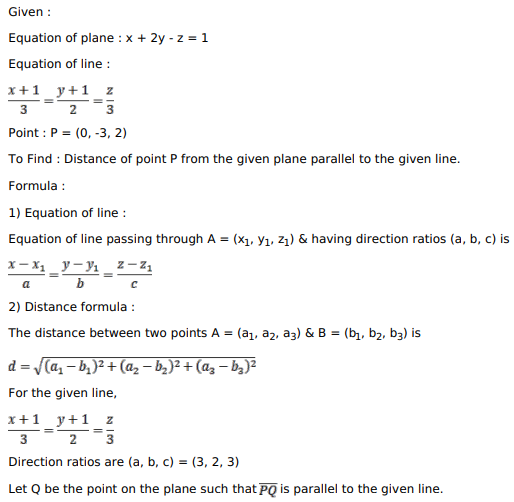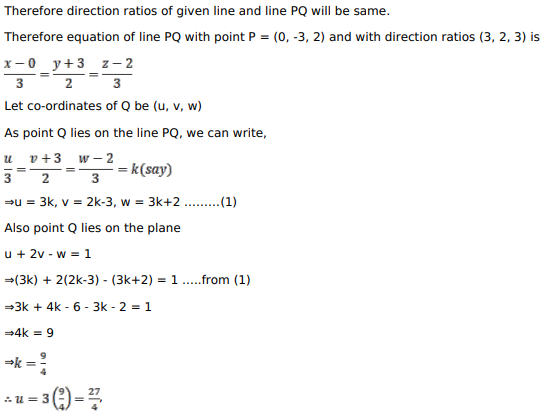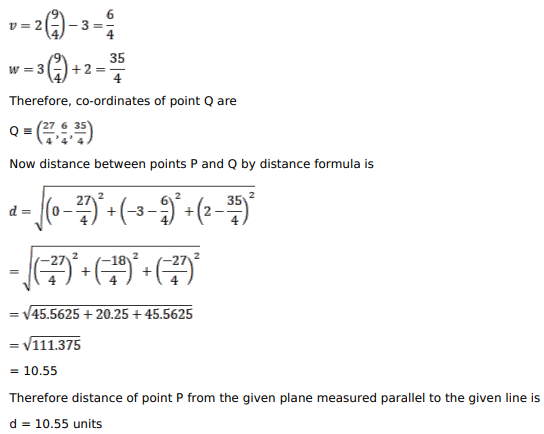# Find the distance of the pointQuestion:

Find the distance of the point $(0,-3,2)$ from the plane $x+2 y-z=1$, measured parallel to the line $\frac{x-1}{3}=\frac{y-1}{2}=\frac{z}{3}$.

Solution: